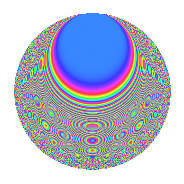# Properties

 Label 804.2.jLevel 804 Weight 2 Character orbit j Rep. character $$\chi_{804}(499,\cdot)$$ Character field $$\Q(\zeta_{6})$$ Dimension 136 Newforms 2 Sturm bound 272 Trace bound 3

# Related objects

## Defining parameters

 Level: $$N$$ = $$804 = 2^{2} \cdot 3 \cdot 67$$ Weight: $$k$$ = $$2$$ Character orbit: $$[\chi]$$ = 804.j (of order $$6$$ and degree $$2$$) Character conductor: $$\operatorname{cond}(\chi)$$ = $$268$$ Character field: $$\Q(\zeta_{6})$$ Newforms: $$2$$ Sturm bound: $$272$$ Trace bound: $$3$$ Distinguishing $$T_p$$: $$7$$

## Dimensions

The following table gives the dimensions of various subspaces of $$M_{2}(804, [\chi])$$.

Total New Old
Modular forms 280 136 144
Cusp forms 264 136 128
Eisenstein series 16 0 16

## Trace form

 $$136q - 4q^{4} + 136q^{9} + O(q^{10})$$ $$136q - 4q^{4} + 136q^{9} + 12q^{10} + 12q^{13} + 20q^{14} - 4q^{16} - 48q^{20} - 8q^{21} - 44q^{22} + 12q^{24} - 136q^{25} - 20q^{26} - 6q^{28} - 16q^{29} - 24q^{30} + 30q^{32} - 4q^{36} + 24q^{37} - 18q^{38} + 36q^{40} - 36q^{44} + 48q^{46} - 92q^{49} + 18q^{50} - 28q^{56} + 24q^{60} + 12q^{61} - 68q^{62} + 32q^{64} + 68q^{68} + 12q^{73} - 42q^{74} + 28q^{76} - 8q^{77} - 18q^{78} + 30q^{80} + 136q^{81} - 52q^{82} - 8q^{84} - 54q^{86} - 28q^{88} + 12q^{90} + 20q^{92} - 4q^{93} + 36q^{97} - 102q^{98} + O(q^{100})$$

## Decomposition of $$S_{2}^{\mathrm{new}}(804, [\chi])$$ into irreducible Hecke orbits

Label Dim. $$A$$ Field CM Traces $q$-expansion
$$a_2$$ $$a_3$$ $$a_5$$ $$a_7$$
804.2.j.a $$68$$ $$6.420$$ None $$0$$ $$-68$$ $$0$$ $$4$$
804.2.j.b $$68$$ $$6.420$$ None $$0$$ $$68$$ $$0$$ $$-4$$

## Decomposition of $$S_{2}^{\mathrm{old}}(804, [\chi])$$ into lower level spaces

$$S_{2}^{\mathrm{old}}(804, [\chi]) \cong$$ $$S_{2}^{\mathrm{new}}(268, [\chi])$$$$^{\oplus 2}$$